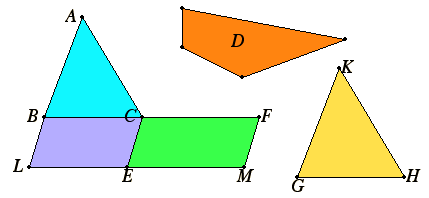# Proposition 25

To construct a figure similar to one given rectilinear figure and equal to another.

Let ABC be the given rectilinear figure to which the figure to be constructed must be similar, and D that to which it must be equal.

It is required to construct one figure similar to ABC and equal to D.

Let there be applied to BC the parallelogram BE equal to the triangle ABC, and to CE the parallelogram CM equal to D in the angle FCE which equals the angle CBL.Then BC is in a straight line with CF, and LE with EM.

Take a mean proportional GH to BC and CF, and describe KGH similar and similarly situated to ABC on GH.

V.19,Cor

Then, since BC is to GH as GH is to CF, and, if three straight lines are proportional, then the first is to the third as the figure on the first is to the similar and similarly situated figure described on the second, therefore BC is to CF as the triangle ABC is to the triangle KGH.

VI.1

But BC is to CF as the parallelogram BE is to the parallelogram EF.

Therefore also the triangle ABC is to the triangle KGH as the parallelogram BE is to the parallelogram EF. Therefore, alternately, the triangle ABC is to the parallelogram BE as the triangle KGH is to the parallelogram EF.

V.Def.5

But the triangle ABC equals the parallelogram BE, therefore the triangle KGH also equals the parallelogram EF. And the parallelogram EF equals D, therefore KGH also equals D.

And KGH is also similar to ABC. Therefore this figure KGH has been constructed similar to the given rectilinear figure ABC and equal to the other given figure D.

Q.E.F.

## Guide

This proposition solves a similar problem, to find a figure with the size of one figure but the shape of another, a problem reputedly solved by Pythagoras. It is used in the proofs of propositions VI.28 and VI.29